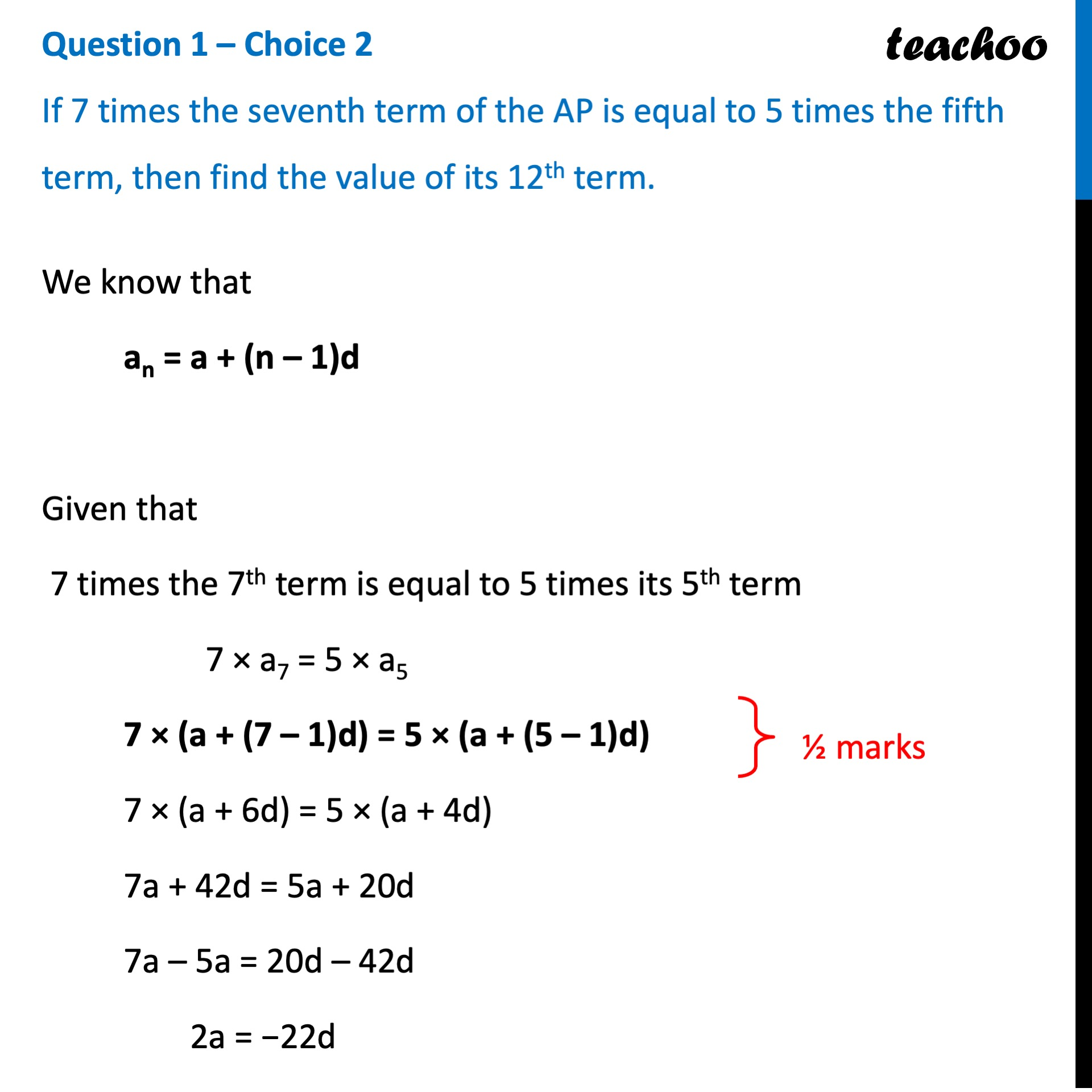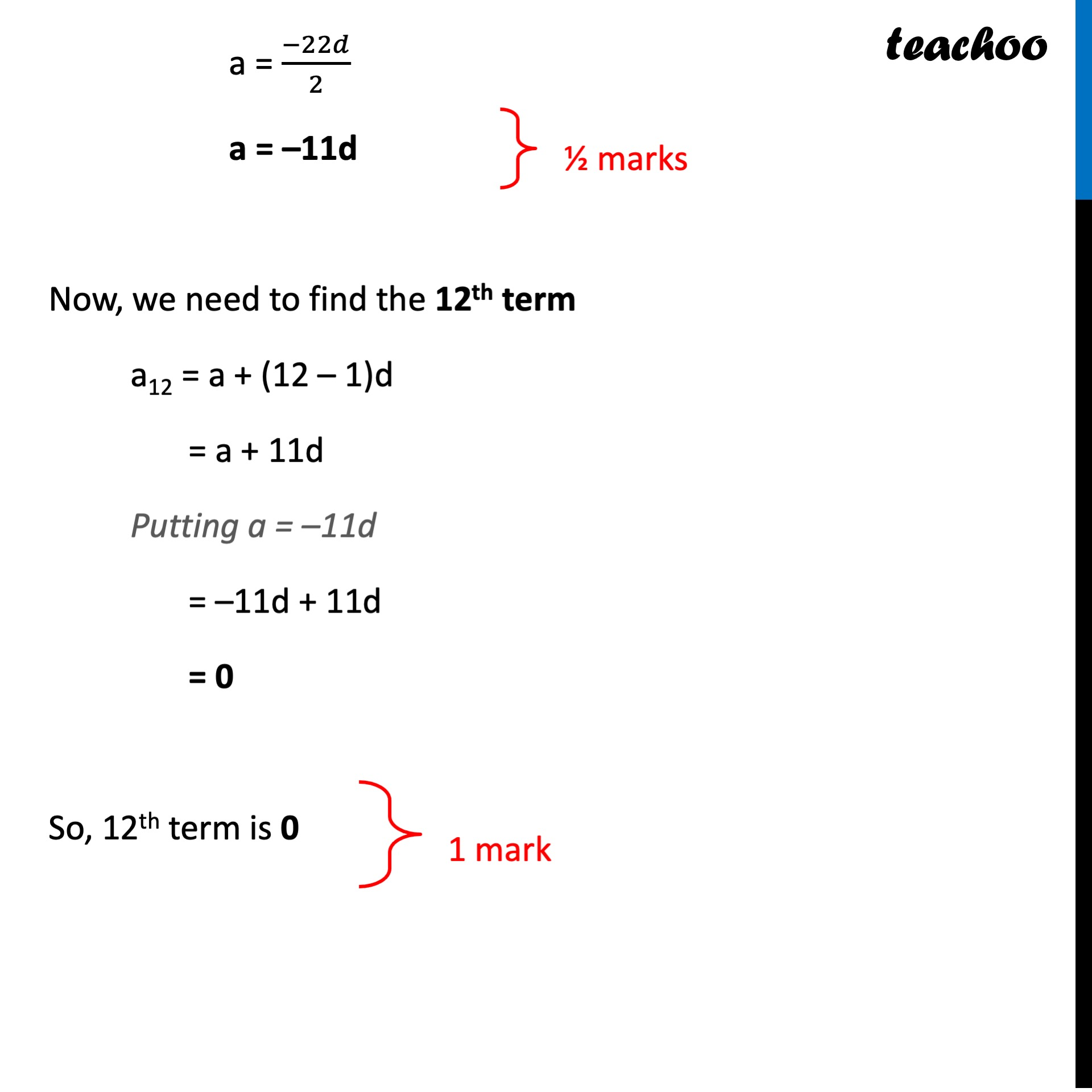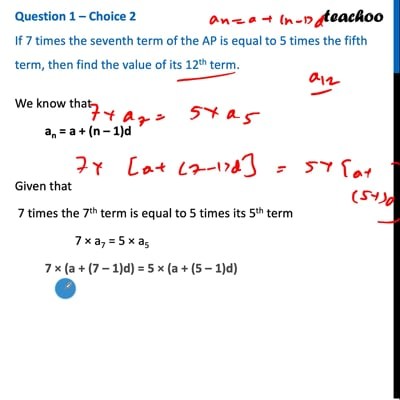CBSE Class 10 Sample Paper for 2022 Boards - Maths Standard [Term 2]

Class 10
Solutions of Sample Papers for Class 10 Boards

## If 7 times the seventh term of the AP is equal to 5 times of the fifth term, then find the value of its 12 th term.

This question is similar to Question 12 or 2nd CBSE Class 10 Sample Paper 2020 BoardsQuestion 1 – Choice 2

If 7 times the seventh term of the AP is equal to 5 times the fifth term, then find the value of its 12th term.This video is only available for Teachoo black users

Introducing your new favourite teacher - Teachoo Black, at only ₹83 per month

### Transcript

Question 1 – Choice 2 If 7 times the seventh term of the AP is equal to 5 times the fifth term, then find the value of its 12th term. We know that an = a + (n – 1)d Given that 7 times the 7th term is equal to 5 times its 5th term 7 × a7 = 5 × a5 7 × (a + (7 – 1)d) = 5 × (a + (5 – 1)d) 7 × (a + 6d) = 5 × (a + 4d) 7a + 42d = 5a + 20d 7a – 5a = 20d – 42d 2a = −22d a = (−22𝑑)/2 a = –11d Now, we need to find the 12th term a12 = a + (12 – 1)d = a + 11d Putting a = –11d = –11d + 11d = 0 So, 12th term is 0